# Graphing Linear Equations 1 Equations tables of values

• Slides: 17Graphing Linear Equations 1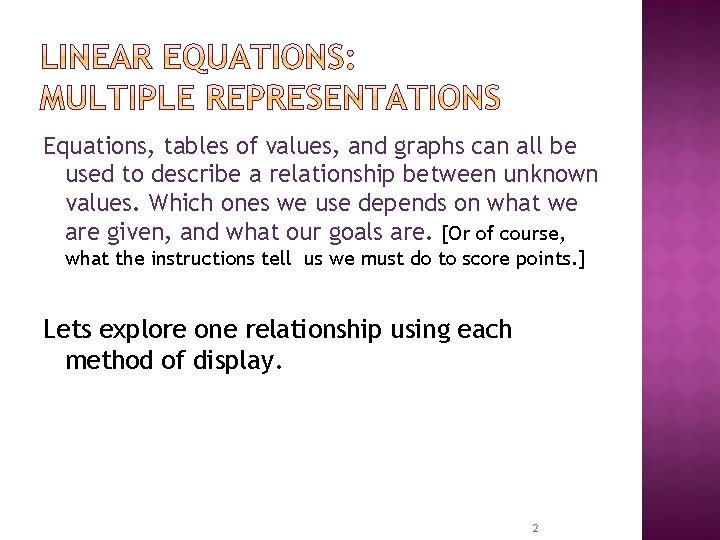Equations, tables of values, and graphs can all be used to describe a relationship between unknown values. Which ones we use depends on what we are given, and what our goals are. [Or of course, what the instructions tell us we must do to score points. ] Lets explore one relationship using each method of display. 2Your Mom is pulling out all the stops in an effort to get you to work to your full potential in school. She offers you a deal. She will give you \$20 if you have at least a B average for the first marking period, plus an extra \$5 for every A you earn. Let’s assume that you are willing to put forth the effort (because that is all it really takes!) and you do have an average of at least a B. We can figure out how much money you can pocket each marking period. 3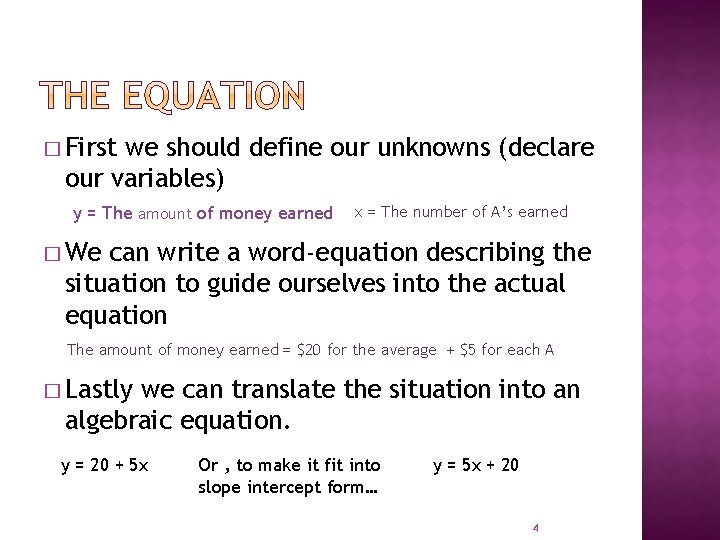� First we should define our unknowns (declare our variables) y = The amount of money earned x = The number of A’s earned � We can write a word-equation describing the situation to guide ourselves into the actual equation The amount of money earned = \$20 for the average + \$5 for each A � Lastly we can translate the situation into an algebraic equation. y = 20 + 5 x Or , to make it fit into slope intercept form… y = 5 x + 20 4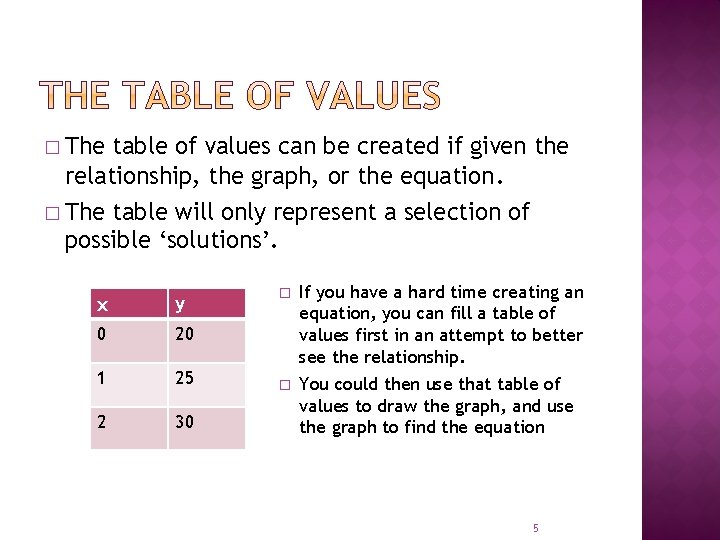� The table of values can be created if given the relationship, the graph, or the equation. � The table will only represent a selection of possible ‘solutions’. x y 0 20 1 25 2 30 � If you have a hard time creating an equation, you can fill a table of values first in an attempt to better see the relationship. � You could then use that table of values to draw the graph, and use the graph to find the equation 5� You can create the graph simply from a table of values. Each row represents a coordinate pair. { (0, 20), (1, 25), (2, 30) } x y 0 20 1 25 2 30 y 40 30 20 10 x 2 4 6 8 Every point on the line shows a possible solution. (6, 45) represents 6 A’s earning \$45. 6� The slope of the line is a measurement that describes the steepness of a line. �If the line slants down from left to right the slope is negative § If the line slants up from left to right the slope is positive. § The closer the slope is to zero, the closer the line is to horizontal. [horizontal lines have a slope of 0] § The closer the slope gets to ±∞, the closer the line is to vertical. [vertical lines have an undefined slope] 7� The y-intercept is the value of y when x=0. The y-intercept is the value of y when the line crosses the y-axis. The y-intercept is the ‘b’ in slope intercept form ( y = mx + b) � The y-intercept. x-intercept The x-intercept is the value of x when y=0. The x-intercept is the value of x when the line crosses the y-axis. The time we most often find the x-intercept is when we want to graph but are given an equation that is not easily transformed into slope intercept form. 8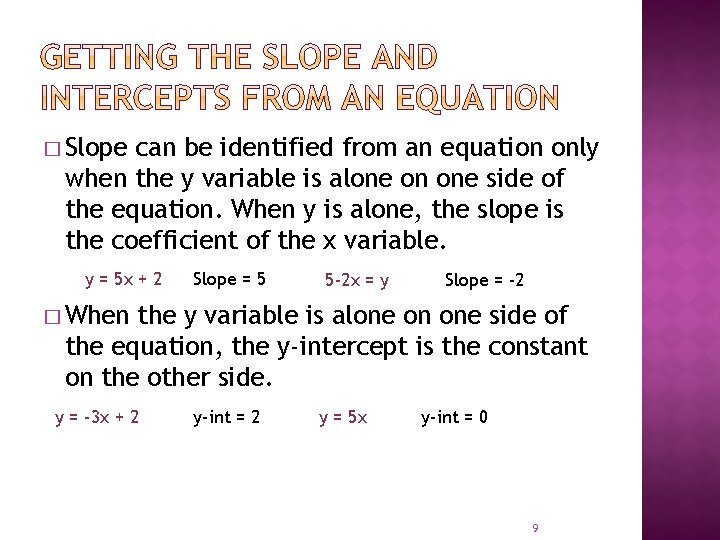� Slope can be identified from an equation only when the y variable is alone on one side of the equation. When y is alone, the slope is the coefficient of the x variable. y = 5 x + 2 Slope = 5 5 -2 x = y Slope = -2 � When the y variable is alone on one side of the equation, the y-intercept is the constant on the other side. y = -3 x + 2 y-int = 2 y = 5 x y-int = 0 9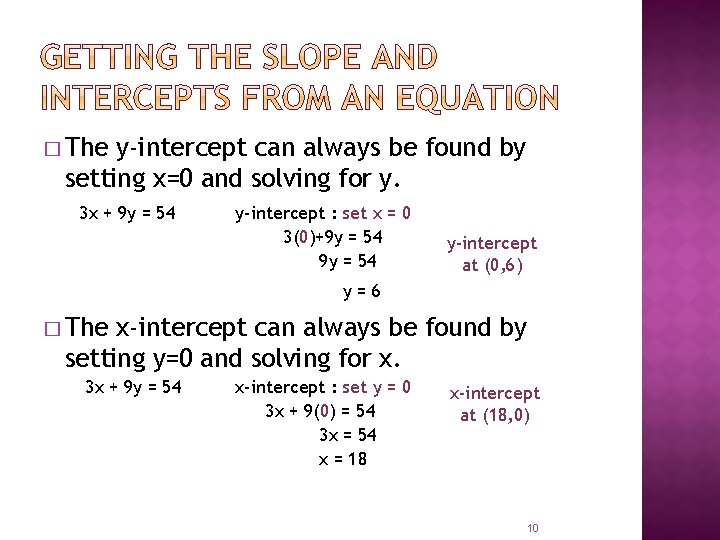� The y-intercept can always be found by setting x=0 and solving for y. 3 x + 9 y = 54 y-intercept : set x = 0 3(0)+9 y = 54 y-intercept at (0, 6) y=6 � The x-intercept can always be found by setting y=0 and solving for x. 3 x + 9 y = 54 x-intercept : set y = 0 3 x + 9(0) = 54 3 x = 54 x = 18 x-intercept at (18, 0) 10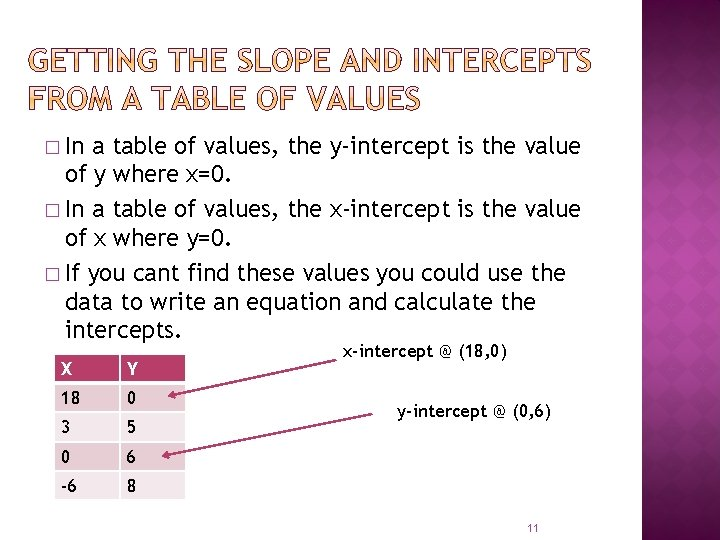� In a table of values, the y-intercept is the value of y where x=0. � In a table of values, the x-intercept is the value of x where y=0. � If you cant find these values you could use the data to write an equation and calculate the intercepts. X Y 18 0 3 5 0 6 -6 8 x-intercept @ (18, 0) y-intercept @ (0, 6) 11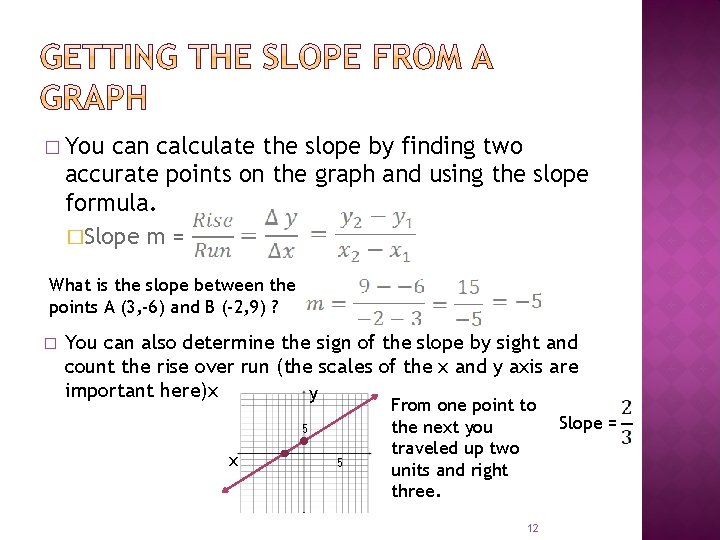� You can calculate the slope by finding two accurate points on the graph and using the slope formula. �Slope m = What is the slope between the points A (3, -6) and B (-2, 9) ? � You can also determine the sign of the slope by sight and count the rise over run (the scales of the x and y axis are important here)x y 5 x 5 From one point to the next you traveled up two units and right three. 12 Slope =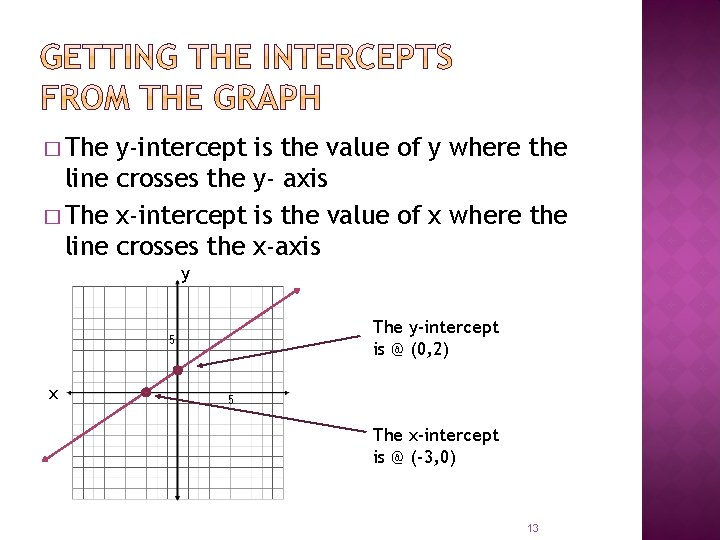� The y-intercept is the value of y where the line crosses the y- axis � The x-intercept is the value of x where the line crosses the x-axis y The y-intercept is @ (0, 2) 5 x 5 The x-intercept is @ (-3, 0) 13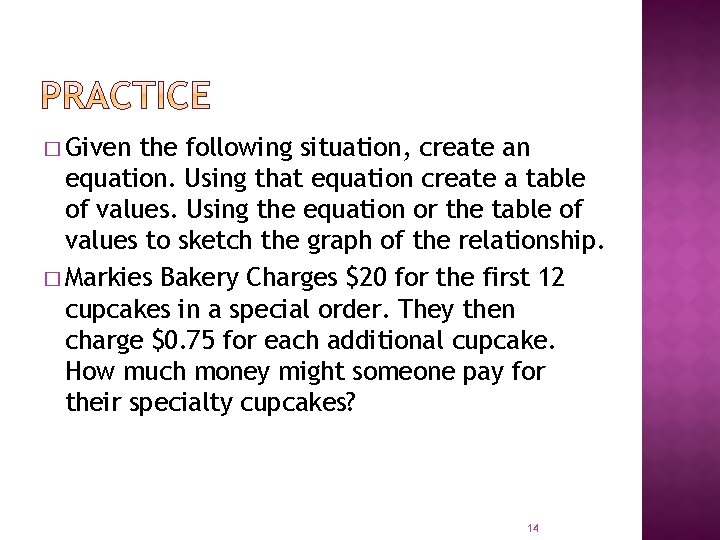� Given the following situation, create an equation. Using that equation create a table of values. Using the equation or the table of values to sketch the graph of the relationship. � Markies Bakery Charges \$20 for the first 12 cupcakes in a special order. They then charge \$0. 75 for each additional cupcake. How much money might someone pay for their specialty cupcakes? 14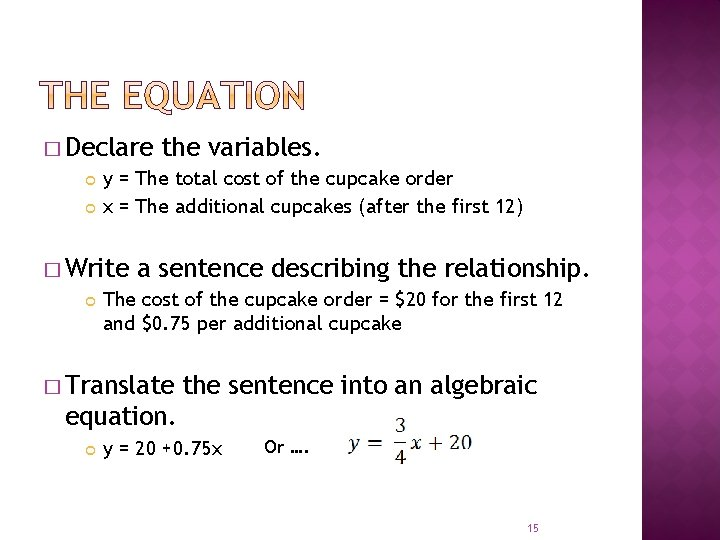� Declare y = The total cost of the cupcake order x = The additional cupcakes (after the first 12) � Write the variables. a sentence describing the relationship. The cost of the cupcake order = \$20 for the first 12 and \$0. 75 per additional cupcake � Translate the sentence into an algebraic equation. y = 20 +0. 75 x Or …. 15Our equation X Y -4 17 0 20 4 23 8 26 If possible, choose x values that will work easily with the equation. 16y Chose your scale carefully 32 Counting consistently on the x and y axis allows you to count boxes when using slope 24 16 8 x 8 16 24 32 The table of values contains points that would have to be estimated on this scale We can determine slope and yintercept from the equation. Slope = y-intercept @ (0, 20) Plot the point (0, 20) and use the slope to find additional points. 17